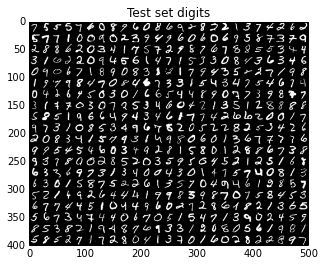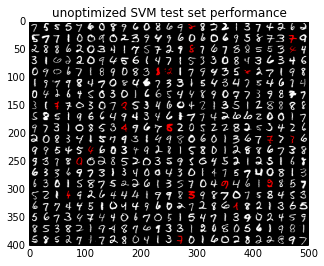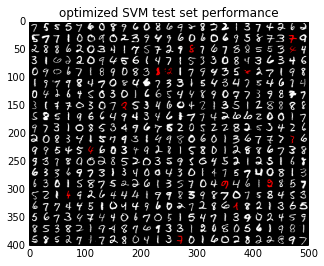# OpenCV: optical character recognition¶

We will use OpenCV (http://www.opencv.org/) for optical character recognition (OCR) using support vector machine (SVM) classifiers. This example is based on OpenCV’s digit tutorial (available in OPENCV_ROOT/samples/python2/digits.py).

import cv2
import numpy as np
import optunity
import optunity.metrics

# comment the line below when running the notebook yourself
%matplotlib inline
import matplotlib.pyplot as plt

# finally, we import some convenience functions to avoid bloating this tutorial
# these are available in the OPTUNITY/notebooks folder
import opencv_utility_functions as util


Load the data, which is available in the OPTUNITY/notebooks directory. This data file is a direct copy from OpenCV’s example.

digits, labels = util.load_digits("opencv-digits.png")

loading "opencv-digits.png" ...


We preprocess the data and make a train/test split.

rand = np.random.RandomState(100)
shuffle = rand.permutation(len(digits))
digits, labels = digits[shuffle], labels[shuffle]

digits2 = map(util.deskew, digits)
samples = util.preprocess_hog(digits2)

train_n = int(0.9*len(samples))

digits_train, digits_test = np.split(digits2, [train_n])
samples_train, samples_test = np.split(samples, [train_n])
labels_train, labels_test = np.split(labels, [train_n])

test_set_img = util.mosaic(25, digits[train_n:])
plt.title('Test set digits')
plt.imshow(test_set_img, cmap='binary_r')

<matplotlib.image.AxesImage at 0x7fd3ee2e4990>Now, it’s time to construct classifiers. We will use a SVM classifier with an RBF kernel, i.e. $$\kappa(\mathbf{u},\mathbf{v}) = \exp(-\gamma\|\mathbf{u}-\mathbf{v}\|^2)$$. Such an SVM has two hyperparameters that must be optimized, namely the misclassification penalty $$C$$ and kernel parameter $$\gamma$$.

We start with an SVM with default parameters, which in this case means: $$C=1$$ and $$\gamma=0.5$$.

model_default = util.SVM()
model_default.train(samples_train, labels_train)
vis_default, err_default = util.evaluate_model(model_default, digits_test, samples_test, labels_test)
plt.title('unoptimized SVM test set performance')
plt.imshow(vis_default)

error: 4.20 %
confusion matrix:
[[50  0  0  0  0  0  0  0  0  0]
[ 0 45  0  0  1  0  0  0  0  0]
[ 0  0 43  1  0  0  0  0  3  1]
[ 0  0  0 48  0  1  0  1  0  0]
[ 0  0  0  0 52  0  2  0  1  1]
[ 0  0  0  0  0 49  0  0  0  0]
[ 0  0  0  0  0  0 48  0  0  0]
[ 0  0  1  3  0  0  0 54  0  0]
[ 0  1  0  0  0  2  0  0 50  0]
[ 1  0  0  0  0  0  0  1  0 40]]

<matplotlib.image.AxesImage at 0x7fd3ee288ad0>Next, we will construct a model with optimized hyperparameters. First we need to build Optunity’s objective function. We will use 5-fold cross-validated error rate as loss function, which we will minimize.

@optunity.cross_validated(x=samples_train, y=labels_train, num_folds=5)
def svm_error_rate(x_train, y_train, x_test, y_test, C, gamma):
model = util.SVM(C=C, gamma=gamma)
model.train(x_train, y_train)
resp = model.predict(x_test)
error_rate = (y_test != resp).mean()
return error_rate


We will use Optunity’s default solver to optimize the error rate given $$0 < C < 5$$ and $$0 < \gamma < 10$$ and up to 50 function evaluations. This may take a while.

optimal_parameters, details, _ = optunity.minimize(svm_error_rate, num_evals=50,
C=[0, 5], gamma=[0, 10])
# the above line can be parallelized by adding pmap=optunity.pmap
# however this is incompatible with IPython

print("Optimal parameters: C=%1.3f, gamma=%1.3f" % (optimal_parameters['C'], optimal_parameters['gamma']))
print("Cross-validated error rate: %1.3f" % details.optimum)

Optimal parameters: C=1.798, gamma=6.072
Cross-validated error rate: 0.025


Finally, we train a model with the optimized parameters and determine its test set performance.

model_opt = util.SVM(**optimal_parameters)
model_opt.train(samples_train, labels_train)
vis_opt, err_opt = util.evaluate_model(model_opt, digits_test, samples_test, labels_test)
plt.title('optimized SVM test set performance')
plt.imshow(vis_opt)

error: 2.80 %
confusion matrix:
[[50  0  0  0  0  0  0  0  0  0]
[ 0 45  0  0  1  0  0  0  0  0]
[ 0  0 44  1  0  0  0  1  1  1]
[ 0  0  0 49  0  0  0  1  0  0]
[ 0  0  1  0 53  0  2  0  0  0]
[ 0  0  0  0  0 49  0  0  0  0]
[ 0  0  0  0  0  0 48  0  0  0]
[ 0  0  2  0  0  0  0 56  0  0]
[ 0  1  0  0  0  1  0  0 51  0]
[ 0  0  0  0  0  0  0  1  0 41]]

<matplotlib.image.AxesImage at 0x7fd3ee4da050>print("Reduction in error rate by optimizing hyperparameters: %1.1f%%" % (100.0 - 100.0 * err_opt / err_default))

Reduction in error rate by optimizing hyperparameters: 33.3%